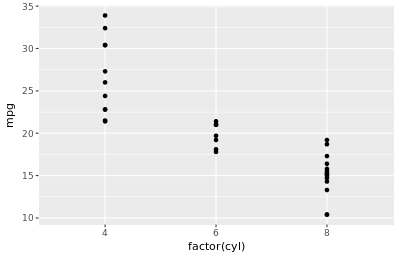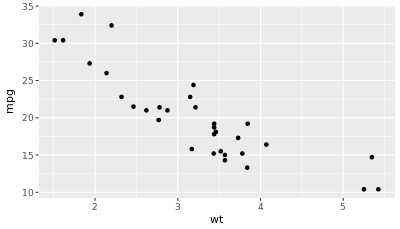#### ggplot(mtcars, aes(x = wt, y = mpg)) + geom_point()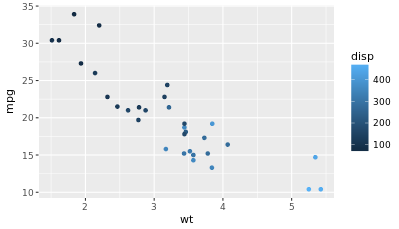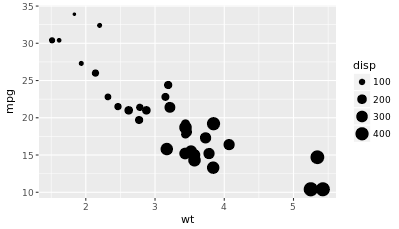#### ggplot(mtcars, aes(x = wt, y = mpg, size = disp)) + geom_point()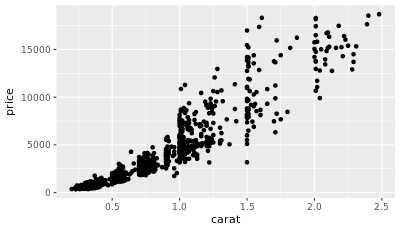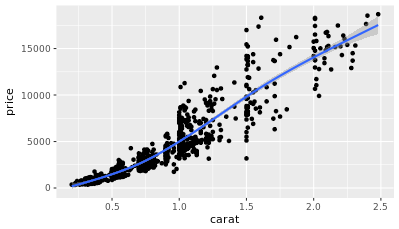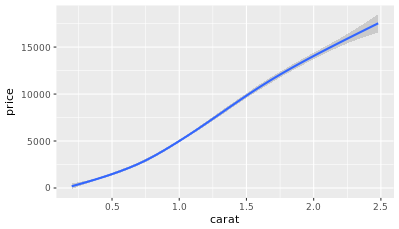#### ggplot(diamonds, aes(x = carat, y = price)) + geom_smooth()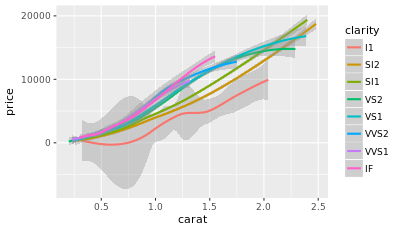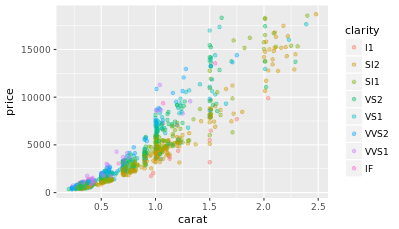### Adding aes() inside geom_point() :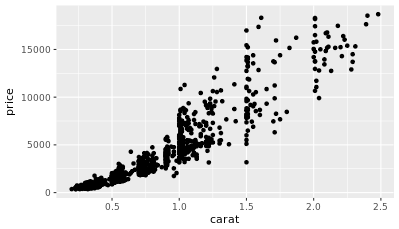#### ggplot(diamonds, aes(x = carat, y = price)) + geom_point(aes(color = clarity))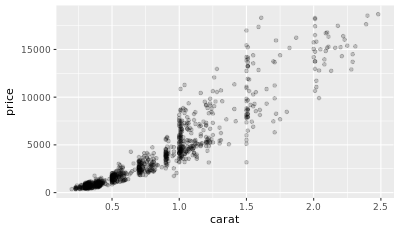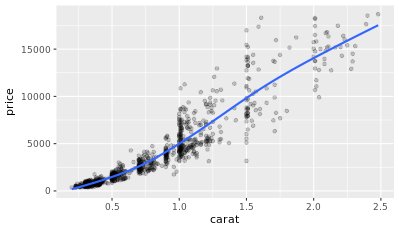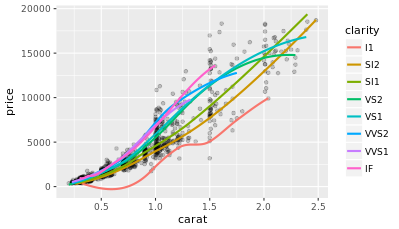#### ggplot(diamonds, aes(x = carat, y = price)) + geom_point(alpha=0.2) + geom_smooth(aes(col = clarity), se = FALSE)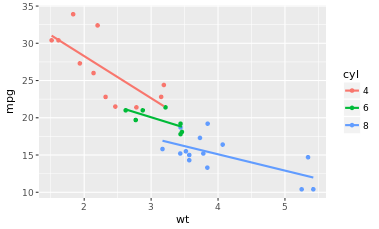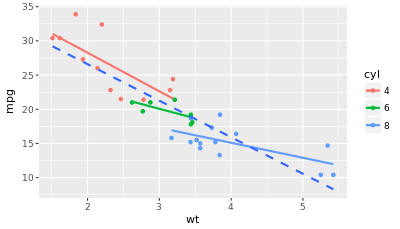#### mtcars\$cyl <- as.factor(mtcars\$cyl) ggplot(mtcars, aes(x = wt, y = mpg, col = cyl)) + geom_point()+ geom_smooth(method='lm', se=FALSE)

Include the lines of the linear models, per cyl.

Note`geom_smooth()` will automatically draw a line per `cyl` subset. It recognizes the groups you want to identify by color in the `aes()` call within the `ggplot()` command.

#### ggplot(mtcars, aes(x = wt, y = mpg, col = cyl)) + geom_point() + geom_smooth(method = "lm", se = FALSE) + geom_smooth(aes(group = 1), method = "lm", se = FALSE, linetype = 2)

Include also a lm for the entire dataset in its whole

Note: the `group=1` aesthetic will tell `ggplot()` to draw a single linear model through all the points.#### mtcars\$cyl <- as.factor(mtcars\$cyl) ggplot(mtcars, aes(x = wt, y = mpg, col = cyl)) + geom_point()+ geom_smooth(method='lm', se=FALSE)

Include the lines of the linear models, per cyl.

Note`geom_smooth()` will automatically draw a line per `cyl` subset. It recognizes the groups you want to identify by color in the `aes()` call within the `ggplot()` command.

#### ggplot(mtcars, aes(x = wt, y = mpg, col = cyl)) + geom_point() + geom_smooth(method = "lm", se = FALSE) + geom_smooth(aes(group = 1), method = "lm", se = FALSE, linetype = 2)

Include also a lm for the entire dataset in its whole

Note: the `group=1` aesthetic will tell `ggplot()` to draw a single linear model through all the points.

``````iris.tidy <- iris %>%
gather(key, Value, -Species) %>%
separate(key, c("Part", "Measure"), "\\.")
``````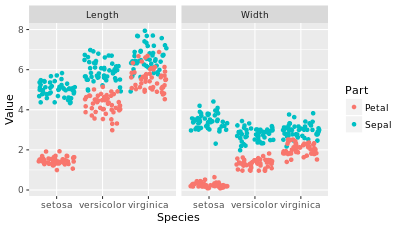``````iris\$Flower <- 1:nrow(iris)
iris.wide <- iris %>%
gather(key, value, -Species, -Flower) %>%
separate(key, c("Part", "Measure"), "\\.") %>%
``````
``` ```
``` ```
``````
``` ```
``` ```
``` ```
``` ```
``` ```
``` Gittering to avoid overplotting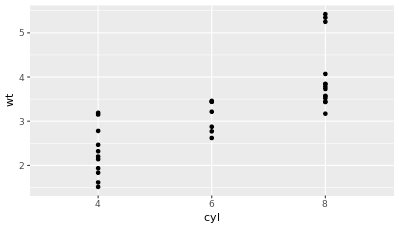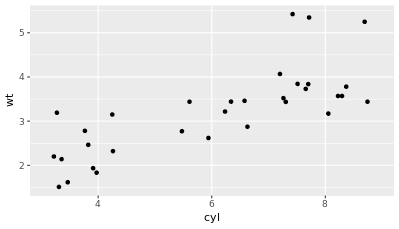Width of the jitter is a bit too wide to be useful.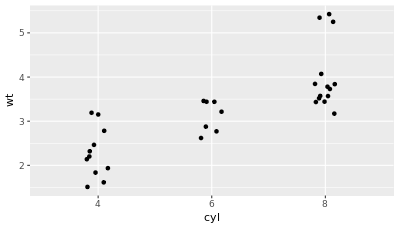Setting the position argument here to position_jitter(0.1), which will set the jittering width directly inside a points layer.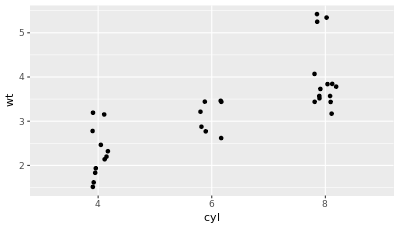ggplot(mtcars, aes(x = cyl, y = wt)) + geom_jitter(width=0.1) ggplot(mtcars, aes(x = cyl, y = wt)) + geom_point(position = position_jitter(0.1)) Note:  jitter can bean argument in geom_point(position = ‘jitter’)a geom itself, geom_jitter()a position function, position_jitter(0.1) ```
``` ```
``` ```
``` ```
``` ```
``` ```
```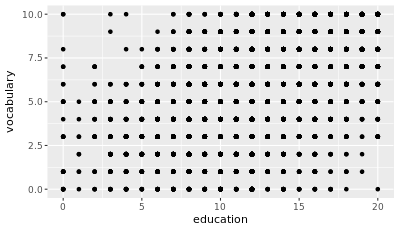ggplot(Vocab, aes(education, vocabulary)) + geom_point()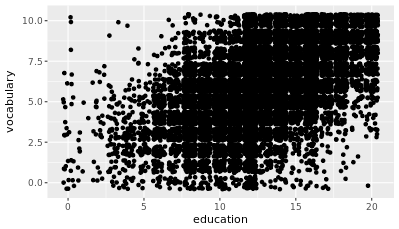ggplot(Vocab, aes(education, vocabulary)) + geom_jitter()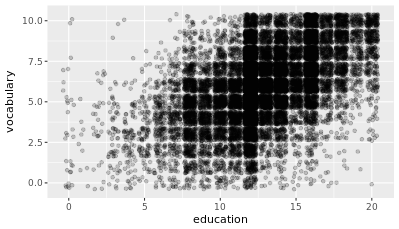ggplot(Vocab, aes(education, vocabulary)) + geom_jitter(alpha=0.2)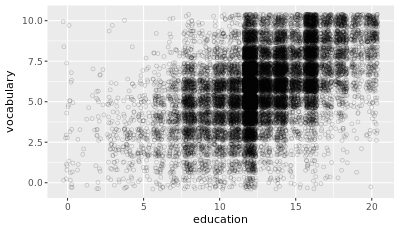ggplot(Vocab, aes(education, vocabulary)) + geom_jitter(alpha=0.2, shape=1)  Note: Jittering and alpha blending serves as a great solution to the overplotting problem here. Setting the shape to 1 didn’t really help, but it was useful in the previous exercises when you had less data. se : logical value. If TRUE, confidence interval is displayed around smooth. ```
``` ```
``` ```
``` ```
``` ```
``` Recent Posts WordPress Resources at SiteGround Hello world! Archives June 2018 June 2020 M T W T F S S « Jun     1234567 891011121314 15161718192021 22232425262728 2930   ©2020 * WebMust var ElementorProFrontendConfig = {"ajaxurl":"https:\/\/webmust.org\/wp-admin\/admin-ajax.php","nonce":"bd472587bb","shareButtonsNetworks":{"facebook":{"title":"Facebook","has_counter":true},"twitter":{"title":"Twitter"},"google":{"title":"Google+","has_counter":true},"linkedin":{"title":"LinkedIn","has_counter":true},"pinterest":{"title":"Pinterest","has_counter":true},"reddit":{"title":"Reddit","has_counter":true},"vk":{"title":"VK","has_counter":true},"odnoklassniki":{"title":"OK","has_counter":true},"tumblr":{"title":"Tumblr"},"delicious":{"title":"Delicious"},"digg":{"title":"Digg"},"skype":{"title":"Skype"},"stumbleupon":{"title":"StumbleUpon","has_counter":true},"telegram":{"title":"Telegram"},"pocket":{"title":"Pocket","has_counter":true},"xing":{"title":"XING","has_counter":true},"whatsapp":{"title":"WhatsApp"},"email":{"title":"Email"},"print":{"title":"Print"}},"facebook_sdk":{"lang":"en_US","app_id":""}}; var elementorFrontendConfig = {"environmentMode":{"edit":false,"wpPreview":false},"is_rtl":false,"breakpoints":{"xs":0,"sm":480,"md":768,"lg":1025,"xl":1440,"xxl":1600},"version":"2.5.16","urls":{"assets":"https:\/\/webmust.org\/wp-content\/plugins\/elementor\/assets\/"},"settings":{"page":[],"general":{"elementor_global_image_lightbox":"yes","elementor_enable_lightbox_in_editor":"yes"}},"post":{"id":881,"title":"ggplot2 - plots","excerpt":""}}; ```